# Jobber "X" (ver. 1.0)

## General information on the Jobber "X"

Note: If you have a Jobber 6 construction calculator manual, the formulas are vertually the same. Only you do not have to hit the "INV" key to enter different modes.

The Jobber X was developed especially for use by anyone connected with the building construction industry.

The Jobber X has 5 different dimensional formats with instant conversion between all formats.

• Feet, inches & fractions
• Decimal (of feet)
• Inches & fractions
• Decimal (of inches)
• Metric (millimeters and meters)

All will work with the many built-in math programs. This means that complex calculations can be made without the use of charts or tables, thus decreasing errors and increasing production.

The primary difference betweeen the Jobber X and other calculators is its use of its special keyboard of 0-15 instead of 0-9. this allows inches and fractions to be entered with a single keystroke each. You will learn about this in a moment.

The Jobber X has 6 different modes

 Mode Key to press 1. Feet, inches & fractions [FIS] 2. Decimal (of feet) [DEC] 3. Metric (millimeters) [MET] 4. Metric (meters) [MET] twice 5. Inches and fractions [INCH] 6. Decimal inches [INCH] twice

Each mode may be interchanged at any time. Thus, you can convert any FIS dimension to Decimal or Metric and vice versa with a single keystroke.

## Understanding Basic Key Definitions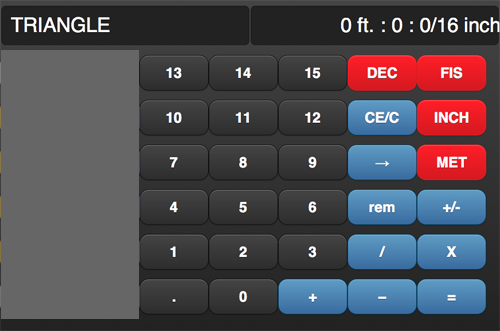These are the basic key functions you will find in each of the Jobber X modes.

• [DEC] - Decimal Feet mode (stardard calculator)
• [FIS] - Feet, Inches, Sixteenths (fractions) mode.
• [INCH] - Inch mode. Press once, becomes inches and fractions. Press twice, becomes decimal inches.
• [MET] - Metric mode. Press once, becomes millimeters. Press twice, becomes meters.
• 0-15 keys are used for entering feet, inches and fractions (see below)
• .,0-9 keys are used for entering decimal feet, decimal inches, meters and millimeters
• +, - , / , X , = .....Addition, subtraction, division, multiplication, equals
• [rem] - Remainder. Displays the remainder value when a FIS dimension is dvided by a whole number
• [→] - Backspace. key is a back space key to fix incorrect entries
• [CE/C] key. When pressed once, clears the entry without loosing any other formulas. When pressed twice, will clear all.

## Entering Dimensions, converting, doing simple math

The main rule when entering dimension in FIS mode is to enter the dimension the same way that you would say it.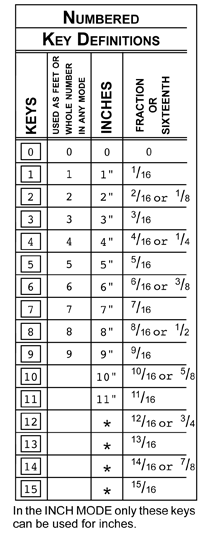While in FIS mode, the display is broken down into feet, inches, and sixteenths. To enter 67 ft, 10, inches and 13/16ths, you would enter it the same way as you would say it. Enter keys     The display will read 67ft: 10: 13/16 From here, you can convert to any format Remarks Conv to Inches Conv to Dec Inches Conv to Dec Feet Conv to Millimeters Conv to Meters Key Pressed [INCH] [INCH] [DEC] [MET] [MET] Display 814: 13/16 inch 814.8125 inch 67.901042 20696.2375 mm 20.696238 mt.
 Now practice entering dimensions as shown on the left and then practice simple simple addition, subtraction, multiplication and division. Remember, enter the dimension exactly as you would say it. Use the keys 10-15 to enter inches and fractions above 10 as shown below. First thing to do is enter FIS mode by pressing the [FIS] button.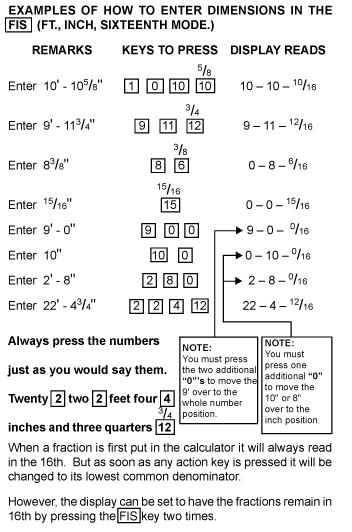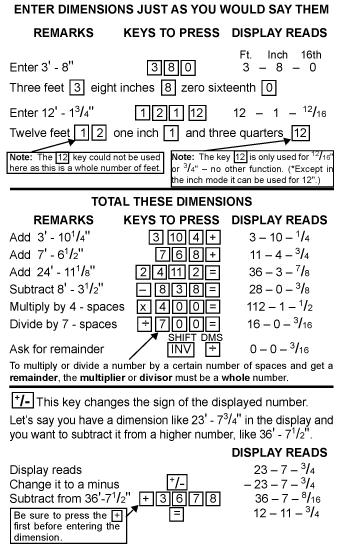It may take a little practice to get use to, but one you do, you will see how much easier and time saving it is entering dimensions.

## Working a Triangle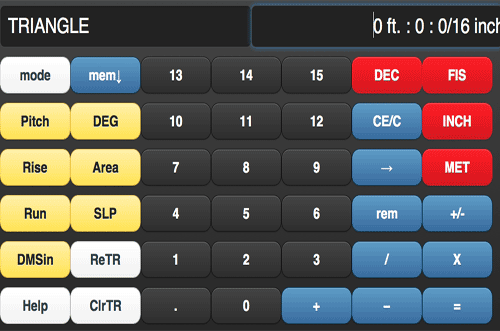Understanding the special triangle function keys Pitch - Bevel or tangent DEG - Degree (Decimal degree. press twice DMS-degrees, minutes, seconds) Rise - Vertical leg Area - Area inside the triangle Run - Base leg ReTR - Recall triangle SLP - Slope leg ClrTR - Clears out all features of a triangle DMSin - Degrees, minutes, seconds input. Help - Help feature of all modes of the Jobber X

A triangle can be easily completed by knowing any 2 given parts: Rise, Run, Slope, Pitch, Tangent or Degree.

The examples below show how to complete a triangle when 2 of the different parts are given. Then it will show the same using millimeters

 The images below show how to solve a triangles with 2 given components.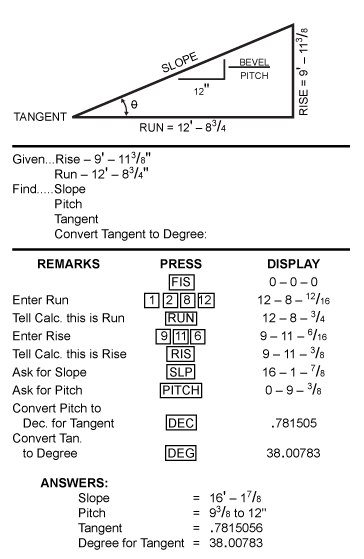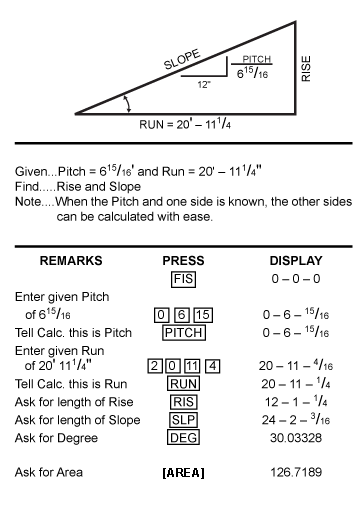Once you are able to solve triangles, squaring up any project is a breeze.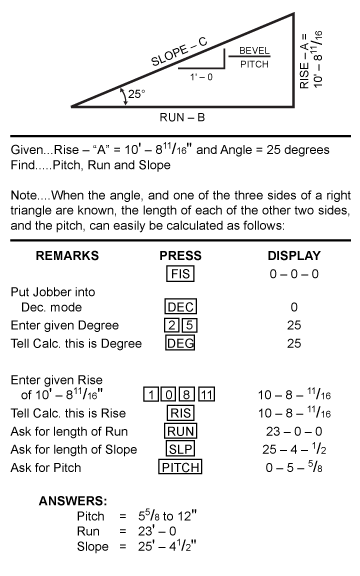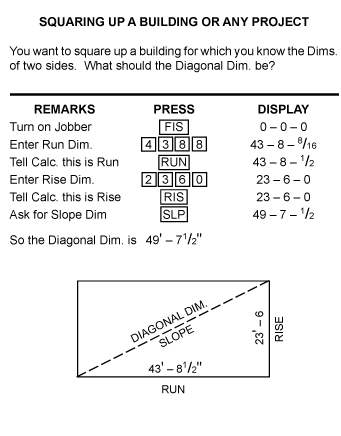Solving a triange in Metric mode. In this case, we are using millimeters. Make sure "mm" is in the display when you press the [MET] button.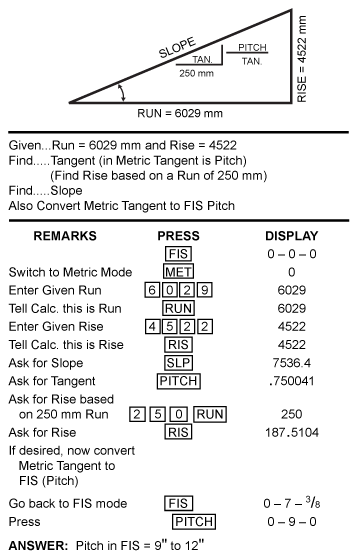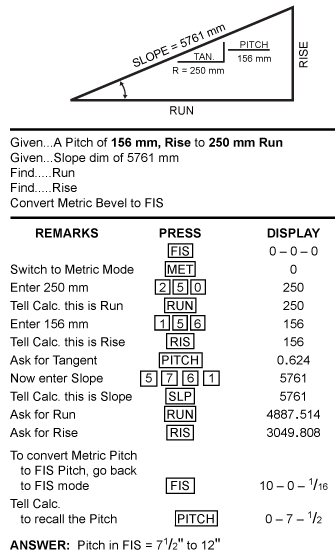## Circle Mode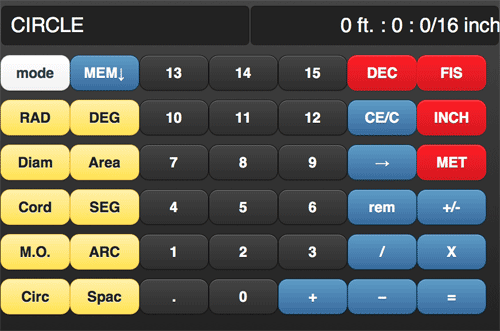Understanding the special circle function keys RAD - Radius DEG - Degree (Decimal degree. press twice DMS-degrees, minutes, seconds) Diam - Diameter Area - Area Cord - Cord SEG - Segment M.O. - Middle Ordinate ARC - Arc Circ - Circumferance Spac - Spacing

 Solving circles easy with the Jobber X. If you know any 2 parts of a circle, the Jobber X will tell you the rest. To activate the circle mode, press the [MODE] button until it says circle in the left hand corner.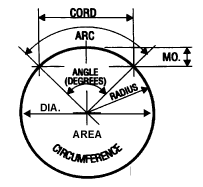Cord and M.O. (Middle Ordinate) Radius and Arc Cord and Degree Middle Ordinate and Degree Cord and Radius or Diameter Circumference - One button gives you the radius Radius and Degree Radius - One button gives you the circumferance Radius and Middle Ordinate

Let solve a circle first with given cord and M.O. (Example 1) and then with a given Radius and degree (Example 2)

Note: If a D.M.S dimension should come up, press the [DEG] key again and it will convert to a decimal degree.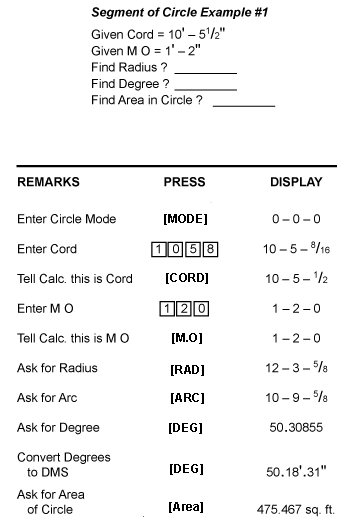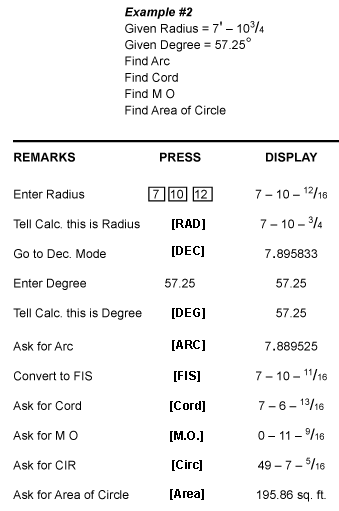Solving bolt circles and bolt patterns of a circle can be solved in seconds.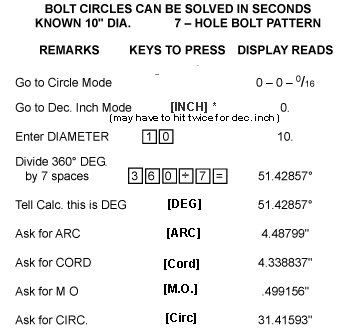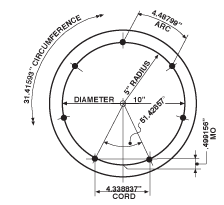Sove a circle using the segmented rise function.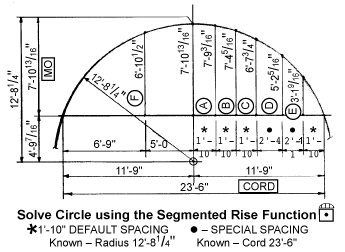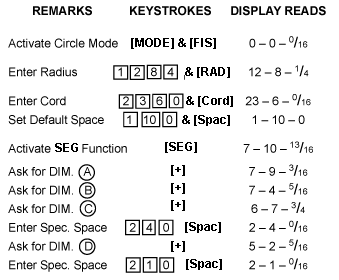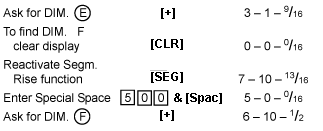Find cubic yard of concrete in this cylinder. Solve one half of a circle using the inch mode.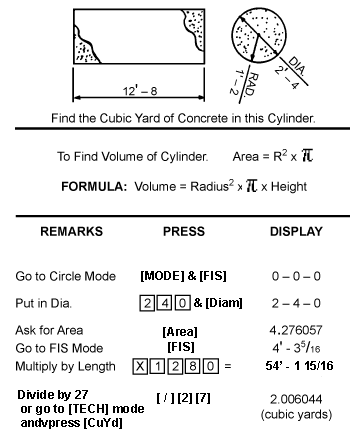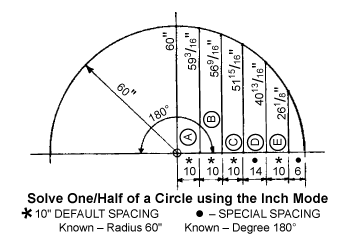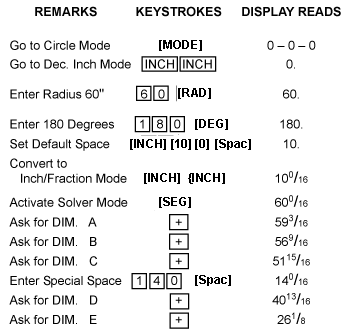## Roof Mode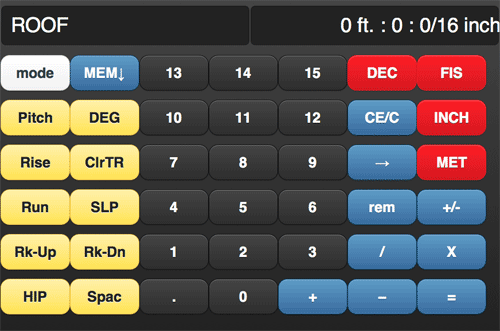Understanding the special Roof function keys Pitch - Pitch or slope Hip - Hip or tangent Rise - Rise or height DEG - Degree (Decimal degree. press twice DMS-degrees, minutes, seconds) Run - Run or base ClrTR - Clears out all entries in triangle Rk-Up - Rake up SLP - Slope Rk-Dn - Rake down Spac - Spacing

The Roof mode works much like the Triangle mode when figuring the rise, run and slope of a triangle. But what the Roof mode adds is frame spacing and hip information as well. The examples below show in detail how to figure these very easily with the Jobber X.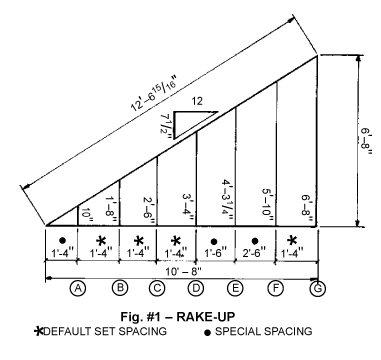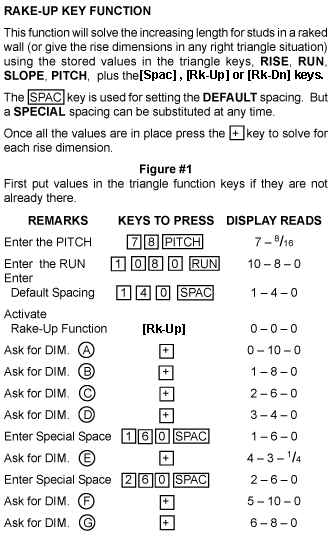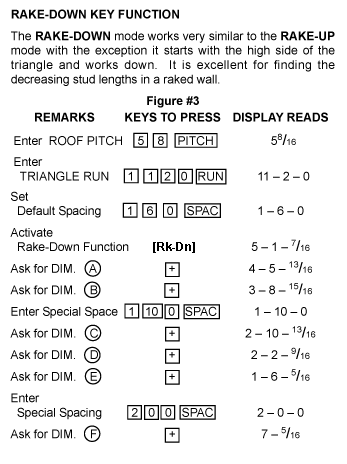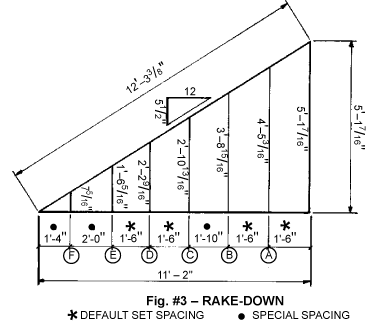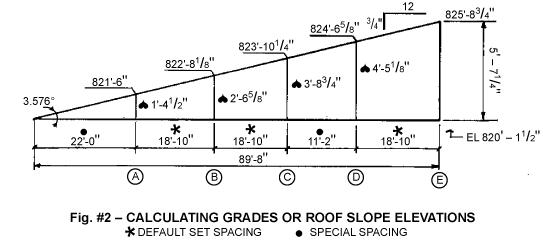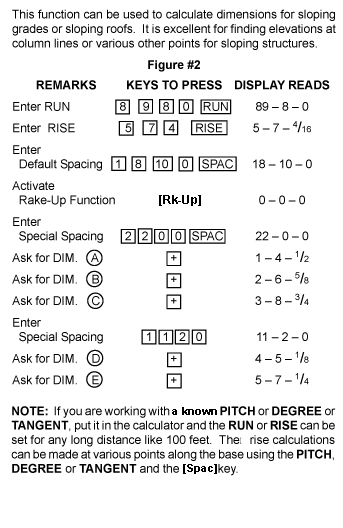## Technical (Tech) Mode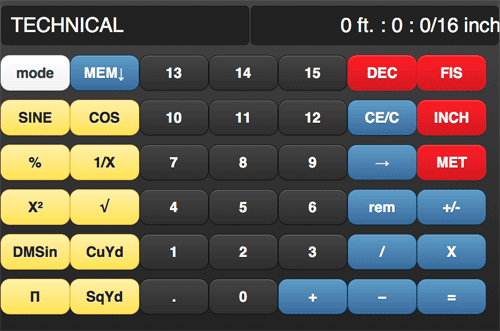Understanding the Technical function keys SINE - Sine (press twice, Invert Sine COS - Consine (press twice, Invert Cosine) % - Percentage key 1/X - Reciprocal or inverse X^2 - Square key √ - Square Root π - pi (3.14.....) CuYd - Cubic yards DMSin - Decimal Degrees (press twice - degrees, minutes, seconds input SqYd - Square yards

## Memories

The Jobber X has 5 permanent memories. These memories will remain there until manually clearing them or refreshing the page. To access these memories, press the MEM button.Once you press this button, the Jobber X will then display 5 areas to place dimensions you want stored and a clear memory key.When you number in the display you want to store, press any of the open memories available. It will then place whatever you had in the display in the selected memory you chose regardless of dimension type.

To recall a number from memory, simply press the memory key you want to restore to the display then perform whatever action you choose.

To Clear a memory, press the RED clear memory button and then press the memory key you would like to clear out. It will then clear that memory to a 0 entry.

All memories will remain while using Jobber X. However, if you refresh the page or reload it, it will clear out all parts of the Jobber X including all stored values.

To hide the memories, simply press the Blue MEM button again.

## Degrees, Minutes, Seconds

Entering and converting DMS is simple with the Jobber X. Press the "DMSin" key. Enter your degree. Press ".". Enter minutes. Press ".". Enter Seconds.

To convert that to a decimal degree, press the "DEG" button. Press it again to toggle back and forth.